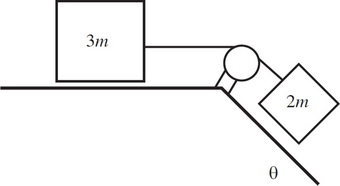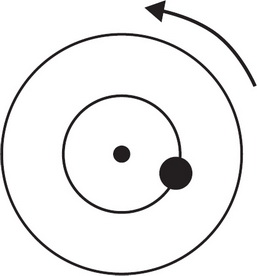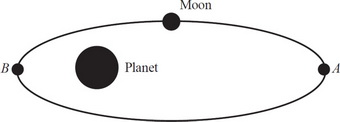# AP Physics 1 Practice Test 7

### Test Information10 questions18 minutes

1. A person is pulling a block of mass m with a force equal to its weight directed 30° above the horizontal plane across a rough surface, generating a friction f on the block. If the person is now pushing downward on the block with the same force 30° above the horizontal plane across the same rough surface, what is the friction on the block? (µk is the coefficient of kinetic friction across the surface.)

2.In the figure above, two blocks of mass 3m and 2m are attached together. The plane is frictionless and the pulley is frictionless and massless. The inclined portion of the plane creates an angle θ with the horizontal floor. What is the acceleration of the block 2m if both blocks are released from rest (gravity = g)?

3. If a roller coaster cart of mass m was not attached to the track, it would still remain in contact with a track throughout a loop of radius R as long as

4.The diagram above shows a top view of an object of mass m on a circular platform of mass 2m that is rotating counterclockwise. Assume the platform rotates without friction. Which of the following best describes an action by the object that will increase the angular speed of the entire system?

5.A moon has an elliptical orbit about the planet as shown above. At point A, the moon has speed vA and is at a distance rA from the planet. At point B, the moon has a speed of vB. Which of the following correctly explains the method for determining the distance of the moon from the planet at point B in the given quantities?

6. A sphere starts from rest atop a hill with a constant angle of inclination and is allowed to roll without slipping down the hill. What force provides the torque that causes the sphere to rotate?

7. Which of the following correctly describes the motion of a real object in free fall? Assume that the object experiences drag force proportional to speed and that it strikes the ground before reaching terminal sped.

8. Which of the following concerning uniform circular motion is true?

9. A girl of mass m and a boy of mass 2m are sitting on opposite sides of a see-saw with its fulcrum in the center. Right now, the boy and girl are equally far from the fulcrum, and it tilts in favor of the boy. Which of the following would NOT be a possible method to balance the see-saw?

10. Given that the Earth’s mass is m, its tangential speed as it revolves around the Sun is v, and the distance from the Sun to the Earth is R, which of the following correctly describes the work done by the centripetal force, Wc, in one year’s time?